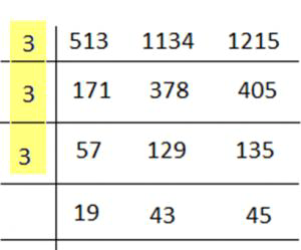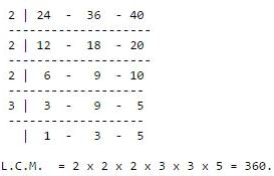# HCF and LCM Questions

09 May 2023

While Preparing for Quantitative aptitude tests, questions on Number system- HCF and LCM are asked frequently.

## Top 10 HCF and LCM Questions

A) 64

B) 42

C) 28

D) 40

A) 9200

B) 9600

C) 9800

D) 9400

A) 40

B) 20

C) 10

D) 30

A) 120

B) 160

C) 220

D) 1

### Q5) H.C.F. of 513, 1134 and 1215 is _____________

A) 18

B) 27

C) 33

D) 36

#### Solution :

We know different methods to calculate H.C.F.

Finding H.C.F. by division method:H.C.F. of 513, 1134 and 1215 = 3 × 3 × 3 = 27.

### Q6) The greatest number which on dividing 1657 and 2037 leaves remainders 6 and 5 respectively, is:

A) 127

B) 305

C) 235

D) 123

Solution :

Required number = H.C.F. of (1657 - 6) and (2037 - 5)

= H.C.F. of 1651 and 2032 = 127.

### Q7) The H.C.F. of two numbers is 23 and the other two factors of their L.C.M. are 13 and 14. The larger of the two numbers is:

A) 276

B) 299

C) 322

D) 345

#### Solution :

Clearly, the numbers are (23 x 13) and (23 x 14).

Larger number = (23 x 14) = 322.

### Q8) HCF of 3/16, 5/12, 7/8 is:

A) 2/47

B) 3/47

C) 1/48

D) 5/48

#### Solution :

HCF of numerators = 1

LCM of denominators = 48

=> 1/48

### Q9) The least number, which when divided by 12, 15, 20 and 54 leaves in each case a remainder of 8 is:

A) 544

B) 548

C) 504

D) 536

#### Solution :

Required number = (L.C.M. of 12, 15, 20, 54) + 8 = 540 + 8 = 548.

### Q10) Find the lowest common multiple of 24, 36 and 40.

A) 120

B) 240

C) 360

D) 480

#### Solution :